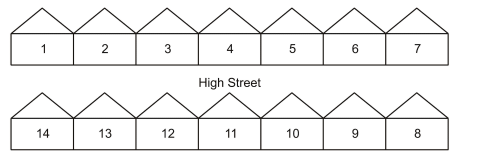# IMAT 2012 Q40 [Houses]

As John walks along the High Street, the numbers to his left increase while the numbers to his right decrease, as in the diagram below.The street where he lives is numbered in the same way. He lives at number 8 Princess Road and the house directly opposite his house is number 11.

How many houses are there on Princess Road?

A. 18
B. 16
C. 20
D. 14
E. 19

Simple steps to solve word problems:

• Underline key information
• Determine what they are trying to ask, and what you will need to solve it
Eliminate any non-essential information
• Draw a picture, graph, or equation
In moments of high stress like exam taking, always work with the paper they give you to avoid careless mistakes.
• Solve.

This question is poorly worded and can be confusing at first. The trick to understanding this question is that you must realise that it only wants the number of houses on one side of the street. To realize this, you will notice that if we took the sum of both sides here, you would have way more than the highest possible answer choice, therefore it must be lower.

The best way to solve this problem is to use a drawing. The parameters for this can be found in the sample image given to us in this problem. One side is increasing and the other is decreasing.

As you can see with this simple drawing, we know that there are 18 houses per side. From here we already know the answer.

Therefore the answer is A, 18.

Note this is very similar to IMAT 2011 Q16, use it for more practice